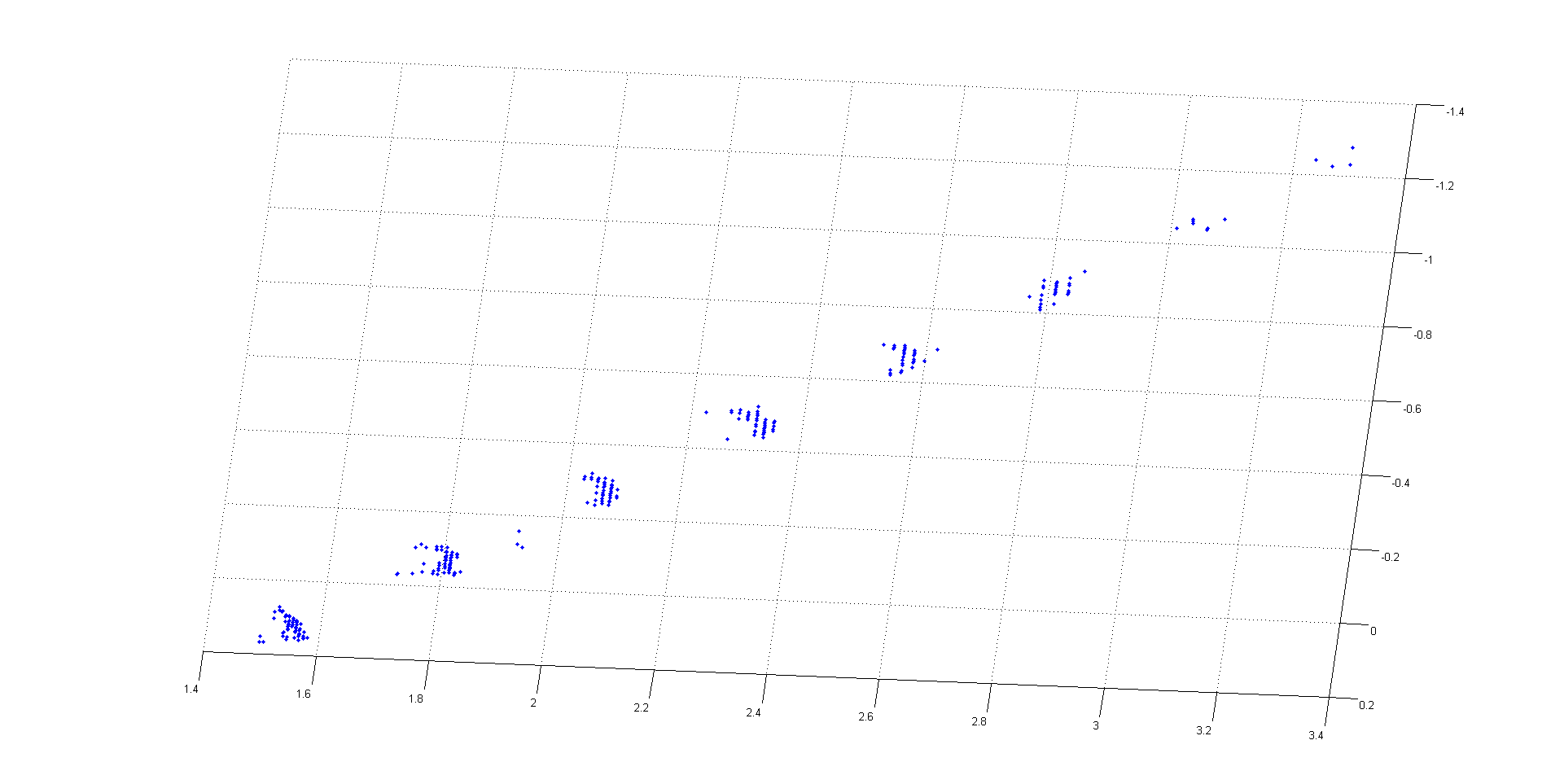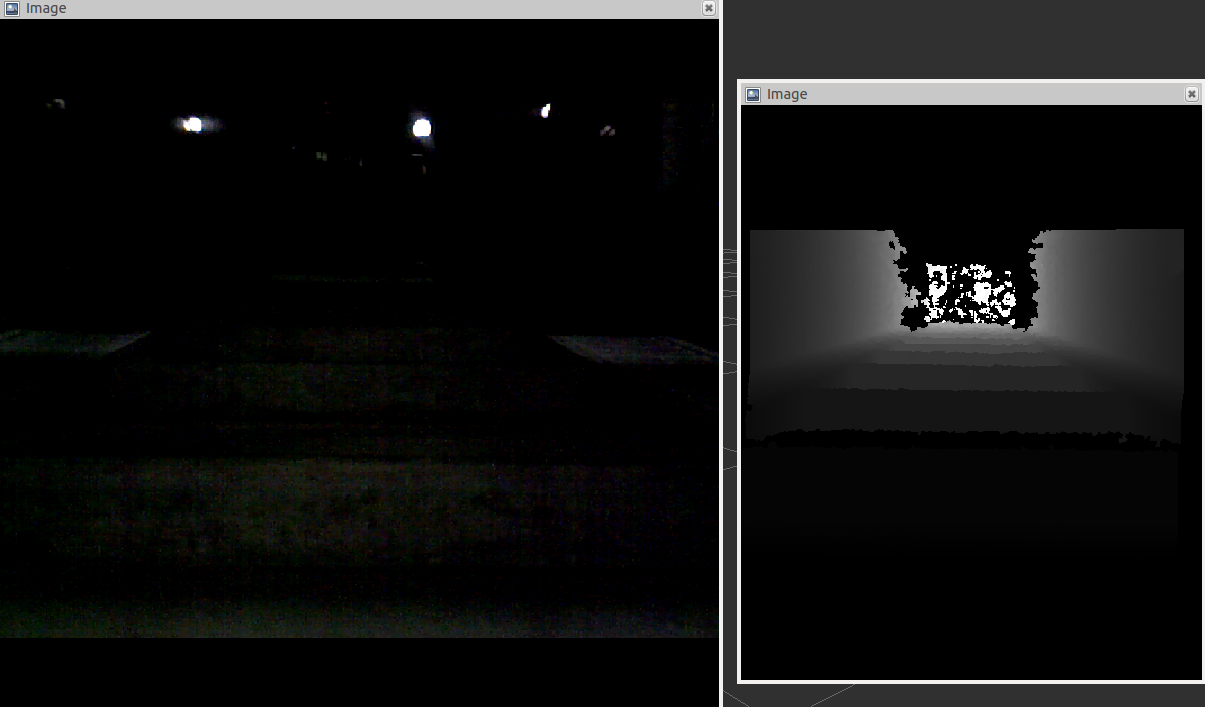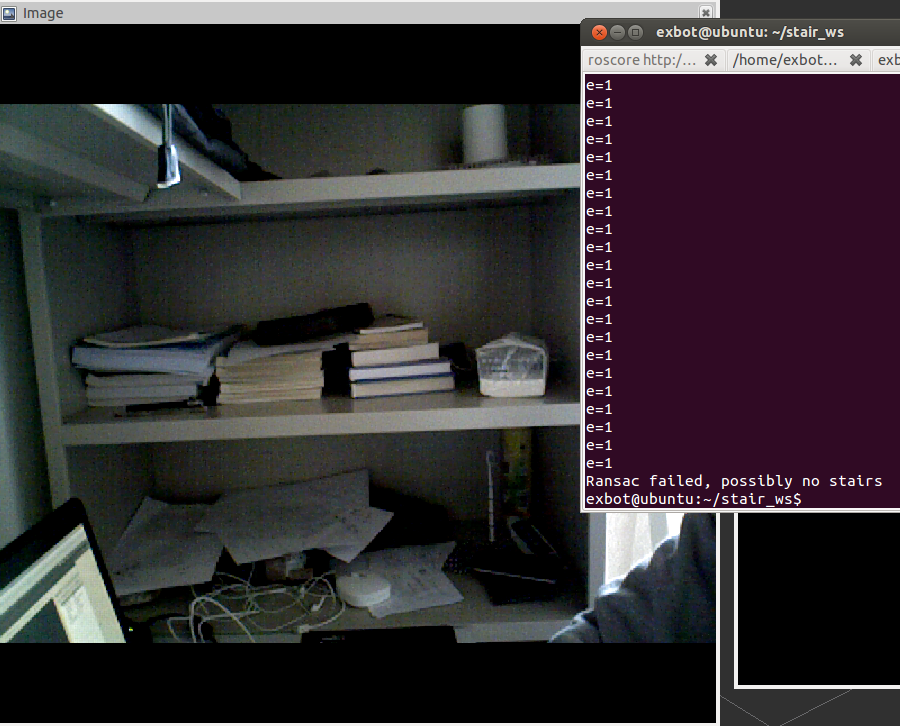# Stair Detection

1.Background

A lot of algorithms used to detect stairways are developed nowadays. Those algorithms all perform very well. However, the precondition for each algorithm is that they should work under different constraints. Some requires a bright environment, while some requires a not too bright environment. The algorithm I developed works even if the environment is dark. In my algorithm, I’ll use only depth information from the RGBD camera to detect the stair. As the point cloud information could be collected regardless of the visible light, the algorithm should work in dark environment.

2.Method

An RGBD camera is used to collect only depth information in this algorithm. The algorithm runs in this way:

First, 100 columns of the image are picked out periodically. Read the Z-axis value of the world coordinate which is constructed by cloud points. Throw away those points which don't have a depth information.Then, calculate the value of 2Z(i)-Z(i-1)-Z(i+1) for each point i. If the value is larger than 20cm, the algorithm push the point into a container, which means it consider the point a feature point where jump happens. A plane is fitted for all the points in the container by RANSAC. In this step a plane is formed, and those points which are not in the plane are excluded. Then, Find all the lines in the plane by RANSAC. According to the direction of the lines, we may figure out the direction of the stair. To calculate the parameters easier, we rotate the stair to X-axis. In the end, we calculate the parameters.
length of the stair is the longest line detected. Height of the stair is the average distance of the lines along Y-axis. Width of the stair is the average distance of the lines along Z-axis. We also find where the first step locates.

I've detected a few objects, and some of them are stairs, some are not. The result are good. It can detect the stairs, and can distinguish stairs from those which are similar to the stairs. The most important is that this algorithm is independent of light which means it could work in dark environment.

3.ExperimentFigure 1: The points remain after the plane is fitted.Figure 2: A special case when the environment is dark. The algorithm also detected the stair. The orientation of the stair is [0.99,0.04,0.1]; the length is 1.52; normvector:15.356; the height is 0.157; 4steps found; the width is 0.55.Figure 3: Algorithm failed when forming a plane, which meant there was no stair.